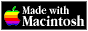# Research Activities of Takashi Hara

Main Theme: Mathematical Physics
(In particular, Rigorous theories of Critical Phenomena and Quantum Field Theory)

I have been studying various critical phenomena, mostly in a mathematicaly rigorous way. Roughly speaking, critical phenomena occurs in a system consisting of (infinitely) many small components, as a result of mutual interaction of the components. This is an interesting topic for Physics, chemistry, and of course for math.

Mathematically, critical phenomena could be seen as representations of some kind of limit theorems. As such, critical phenomena poses a big challenge to probability theory, because interesting critical behaviour can be seen only in systems with strong correlations, where familiar limit theorems (like Central Limit Theorem) are violated.

I have worked on the following different (but mutually related) subjects:

• Classical Spin Systems.
• Self-Avoiding Walks.
• Percolation.
• Lattice Trees and Animals.
• Gravitational collapse.